# Fixed That For You

Someone’s inspirational iterative approach index card wasn’t 100% accurate.Better.

# Honey Cake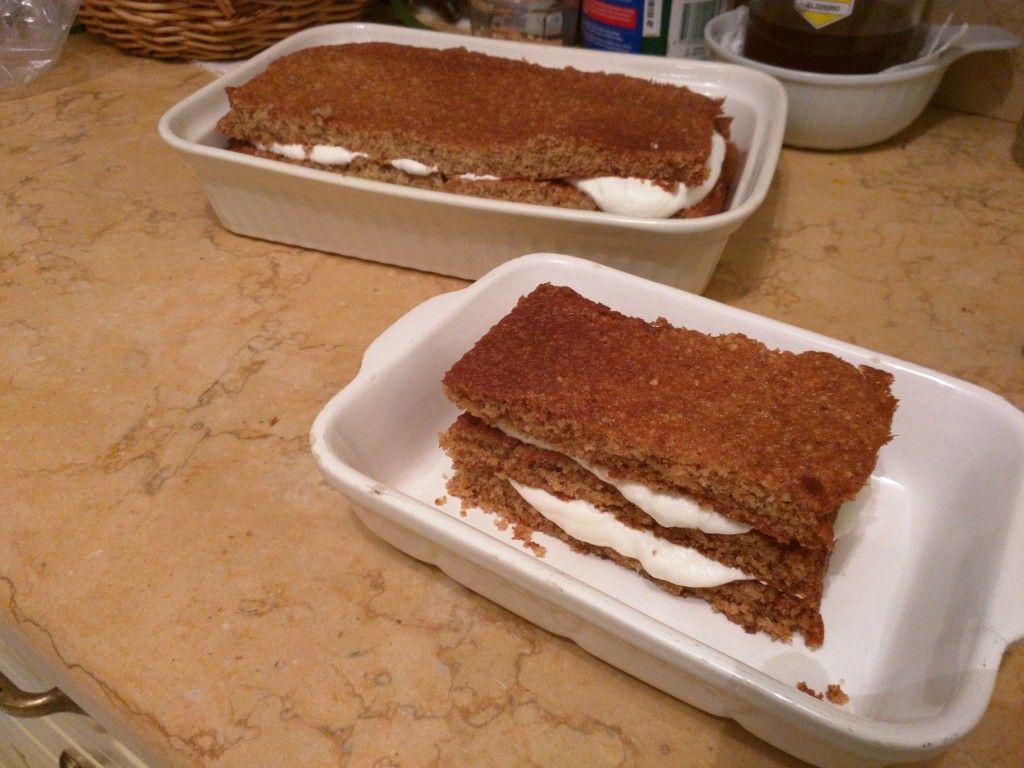Honey Cake

Serves 12 or so?

This lovely cake is very tasty, quick and simple to make, and light but moist.
It was an accidental invention – the first time I made it, I was trying to make biscuits!

Ingredients:

1. 11/2 cups plain flour
2. 1 cup almond meal or “LSA” or almonds ground up in a food processor
3. 1 cup castor sugar
4. 11/2 tsp baking powder
5. 250g butter, softened
6. 2 eggs
7. 1/2 cup honey
8. 75 mL milk
9. 300ml whipping cream

Method:

1. Mix the dry ingredients (A-D) in a bowl,
2. Add everything else except the cream (E-H) and mix well.
3. Line shallow baking trays with baking paper and spread the mixture out thinly.
The recipe covers 2-3 trays.
4. Bake at 180 degrees (170 degrees for fan-forced) for 10-15 minutes, until the edges brown slightly.
5. Cool the layers completely, and cut into even-sized sheets.
6. Whip the cream (I) to stiff peaks.
7. Build the cake in layers, spreading cream between each layer.
It might be easier to make several smaller cakes.

Serve with a hot or cold refreshing drink.

In my previous post, I simulated some mechanical logic gates using a novel 3-bar linkage.
The dimensions of the linkage are critical to achieving correct operation;

Inputs $$\{ false\ false \}$$, $$\{ false\ true \}$$, $$\{ true\ false \}$$ must all produce the same output position.

For the simulations, an empirical approach was used. (fiddle with the values until it looks right)

This time, I’m going to attempt to find the exact values to use for a given stroke, separation distance and output position.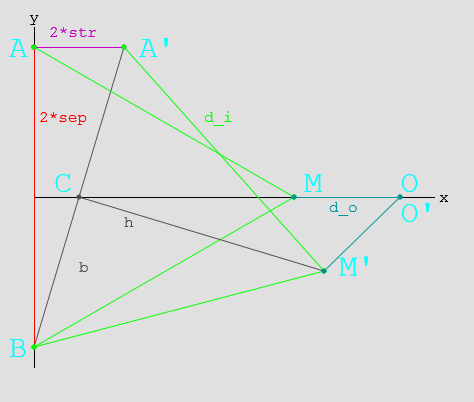Here, you can see the linkage layout for $$\{ false\ false \}$$ and $$\{ true\ false \}$$

# Mathematics

Ahead lurks tough mathematics (which may be unsuitable for liberal-arts majors)
If it’s hard to read, then sorry! It was hard to write!

## Parameters

• $$2 sep$$ Separation between inputs
• $$2 str$$ Stroke length $$false \to true$$
• $$d_i$$ Input link length
• $$d_o$$ Output link length

## Geometry

• $$A(x_a, y_a) = A(0, sep)$$ is the input position when $$A_{state}=false$$
• $$A'(x_a’, y_a’) = A'(2*str, sep)$$ is the input position when $$A_{state}=true$$
• $$B(x_b, y_b) = B(0,-sep)$$ is the input position (The system is symmetric, $$B’$$ does not need to be tested.)
• $$M(x_m, y_m)$$ is the middle position when $$A_{state}=false$$
• $$M'(x_m’, y_m’)$$ is the middle position when $$A_{state}=true$$
• $$O(x_o, y_o)$$ is the output position. ($$O \equiv O’$$ for the gate to function correctly.

## Solving

### Finding $$M$$:

$$M(x_m, y_m) = M( \sqrt{d_i^2 – sep^2}, 0)\\$$

### Finding $$O$$:

\begin{align} O(x_o, y_o) &= O( x_m + d_o, 0 )\\ & = O( \sqrt{d_i^2 – sep^2} + d_o, 0) \end{align}

### Finding $$M’$$:

• $$C'(x_c’, y_c’)$$ is the midpoint of $$BA’$$
• $$b$$ is the length of the line $$BC’$$
• $$h$$ is the length of the line $$C’M’$$
\begin{align} x_c’ &= (x_a’ + x_b)/2 \\ &= str\\ y_c’ &= (y_a’ + y_b)/2 \\ &= 0\\ b &= \sqrt{ sep^2 + str^2 }\\ h &= \sqrt{ d_i^2 – b^2 } && Because BC’ \bot C’M’\\ &= \sqrt{ d_i^2 – sep^2 – str^2 }\\ \end{align}\\

Using this, we can calculate M’:
\begin{align} \frac{h}{b} &= \frac{\sqrt{ d_i^2 – sep^2 – str^2 }}{\sqrt{ sep^2 + str^2 }} \\ &= \sqrt{\frac{ d_i^2 – sep^2 – str^2 }{ sep^2 + str^2 }} \\ &= \sqrt{\frac{d_i^2}{sep^2 + str^2} – 1}\\ \\ x_m’ &= x_c’\ – ( y_c’ – y_b)\frac{h}{b}\\ &= str – \sqrt{\frac{d_i^2}{sep^2 + str^2} – 1} \\ y_m’ &= y_c’ + ( x_c’ – x_b)\frac{h}{b}\\ &= str \sqrt{\frac{d_i^2}{sep^2 + str^2} – 1} \end{align}\\

### Finding $$O’$$:

\begin{align} x_o’ &= x_m’ + \sqrt{ d_o^2 – y_m’^2 }\\ &= ( str – \sqrt{\frac{ d_i^2 }{sep^2 + str^2} – 1}) + \sqrt{ d_o^2 – ( str \sqrt{\frac{d_i^2}{sep^2 + str^2} – 1} )^2 }\\ &= str – \sqrt{\frac{ d_i^2 }{sep^2 + str^2} – 1} + \sqrt{ d_o^2 – str^2 (\frac{d_i^2}{sep^2 + str^2} – 1) }\\ y_o’ &= 0 \end{align} \\

## And then:

Now that $$O$$ and $$O’$$ are found, and we know $$O \equiv O’$$…

### Finding the $$x_o’$$ equation in terms of $$d_o^2$$:

\begin{align} x_o’ &= x_m’ + \sqrt{ d_o^2 – y_m’^2 }\\ d_o^2 – y_m’^2 &= (x_o’ – x_m’)^2 \\ d_o^2 – str^2 (\frac{d_i^2}{sep^2 + str^2} – 1) &= (x_o’ – str + \sqrt{\frac{d_i^2}{sep^2 + str^2} – 1})^2 \\ d_o^2 &= \left( x_o’ – str + \sqrt{\frac{d_i^2}{sep^2 + str^2} – 1} \right)^2 + str^2 (\frac{d_i^2}{sep^2 + str^2} – 1) \\ \end{align}

### Substituting back into the $$x_o$$ equation:

\begin{align} x_o &= \sqrt{d_i^2 – sep^2} + d_o \\ x_o – \sqrt{d_i^2 – sep^2} &= d_o \\ ( x_o – \sqrt{d_i^2 – sep^2} )^2 &= d_o^2 \\ ( x_o – \sqrt{d_i^2 – sep^2} )^2 &= \left( x_o’ – str + \sqrt{\frac{d_i^2}{sep^2 + str^2} – 1} \right)^2 + str^2 (\frac{d_i^2}{sep^2 + str^2} – 1) \end{align}
Ugly, but workable?

# Example:

• $$sep = 50$$ Separation between inputs $$100 mm$$
• $$str = 20$$ Stroke length $$false \to true: 40 mm$$
• $$d_i = ?$$ Input link length
• $$d_o = ?$$ Output link length
• $$x_o = x_o’ = 70$$ Desired output position

\begin{align} ( x_o – \sqrt{d_i^2 – sep^2} )^2 &= \left( x_o’ – str + \sqrt{\frac{d_i^2}{sep^2 + str^2} – 1} \right)^2 + str^2 (\frac{d_i^2}{sep^2 + str^2} – 1) \\ ( 70 – \sqrt{d_i^2 – 50^2} )^2 &= \left( 70 – 20 + \sqrt{\frac{d_i^2}{50^2 + 20^2} – 1} \right)^2 + 20^2 (\frac{d_i^2}{50^2 + 20^2} – 1) \\ \end{align} \\
Pushed through Wolfram Alpha…
\begin{align} \\ d_i &= \sqrt{2900} \\ &= 53.852\\ \text{and}\\ d_o &= x_o – \sqrt{d_i^2 – sep^2} \\ &= 70 – \sqrt{ 2900 – 2500 } \\ &= 50 \end{align}

# Summary:

Phew!

Thusly, one can calculate the input and output linkage lengths for any logic gate.
These dimensions will work for an OR gate too, as the linkages are just reversed.

It’s irksome that I couldn’t work out a symbolic solution for the last equations, especially since $$d_i$$ in the example came out to such a neat value.

I might revisit this further in the future, and it’ll definitely come in handy when building something with these gates.

# Scribing Robot Arm

Development is finally finished, and the campaign has gone live! (And I can talk about it!)

http://www.themostpowerfularm.com/

It’s for a great cause – petitioning the Australian Government to help fund research into Duchenne Muscular Dystrophy.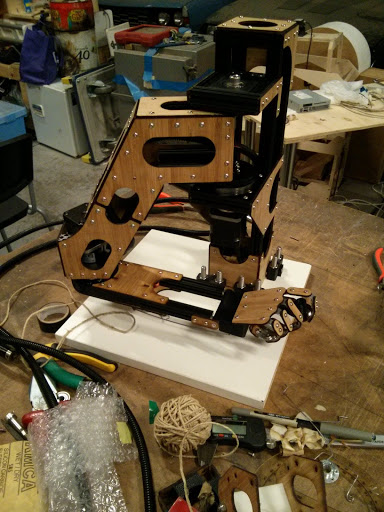The arm in its natural habitat – the RoboDino workshop.

I designed and scratch-built this SCARA-type anatomical robot arm for agencies Finch and Havas, for use in their appeal campaign.

• Stands approximately 350mm tall, with a reach of about 500mm.
• Built from OpenBeam extrusion and laser-cut bamboo.
• Hardware control by an Arduino running grbl with a grblShield v4, and a Micro Maestro servo controller
• Software control by custom software to handle inverse kinematics, font rendering, sequencing
• Queuing service to reliably sequence and write names from campaign site.

The robot arm can draw more than just text, see?

Each short segment in the Hilbert Curve is approximately 1.2mm long! It could draw smaller, but the width of the ink becomes a problem!

A build log is not available yet, but there’s lots of info at the campaign link above, and lots more photos here.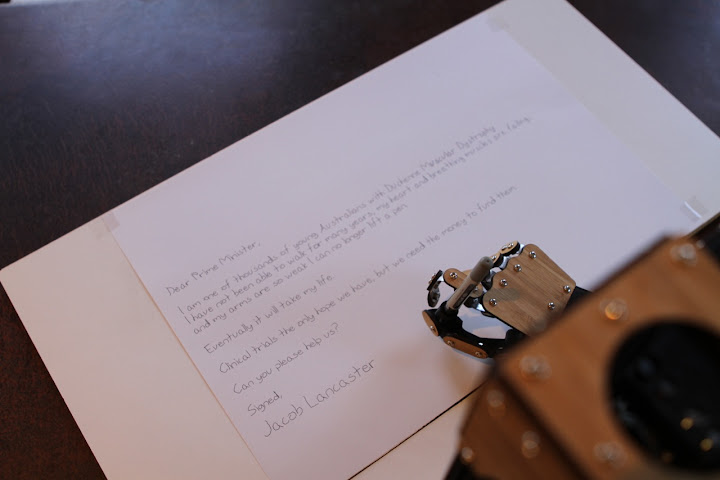Jacob Lancaster’s appeal to the Prime Minister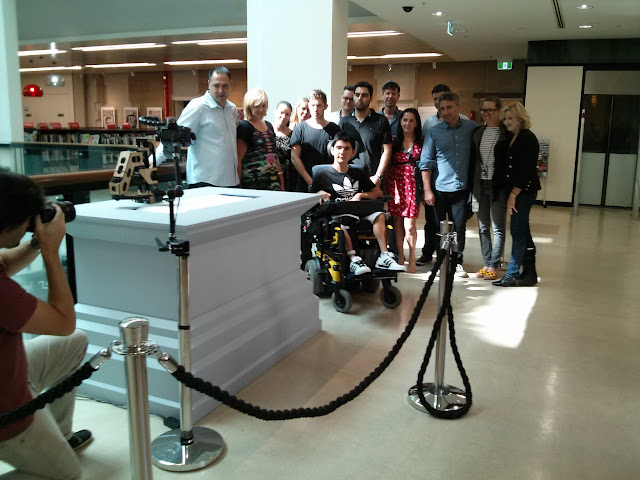Jacob and the team

If you’re in Sydney, you can come and see it in person! It’s at Customs House, Circular Quay, for the next two weeks.

# Finished: Garden Box

Finished another thing! And in miserable weather, too.
Thanks to my wife for doing all the planting, and to my mum for helping with construction and supplying much of the materials as a ‘lots of assembly required’ Christmas present.
It’s a BIG balcony-mounted garden box.Herbs, lettuces, and a chilli plant. All set and ready to grow.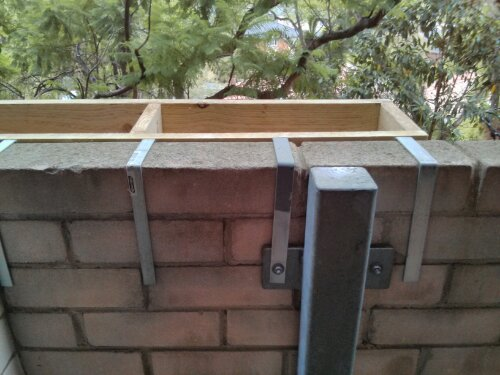Sturdily anchored to the support posts, let’s hope it won’t fall down.1.9 m wide, and built out of treated pine. Note the straps underneath to stop the planters from falling through. (Nobody wants that landing on their head) Also, the EIGHT retaining straps (3mm gal steel) anchoring it to the wall.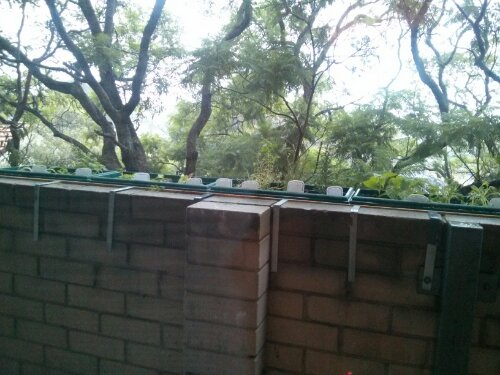They’re enjoying the otherwise miserable weather we’re having!

# On Location64 bits fit neatly onto an 8×8 matrix of black/white squares. Those 64 bits, naively encoding latitude/longitude, will at *worst* locate any point on the planet to within 1cm.

Equatorial circumference: 40,075,017 m
Meridional circumference: 40,007,860 m

Assuming 32 bits per measurement;
Longitudinal precision: 40075017 m / (2^32) in cm = 0.9330692 centimetres
Latitudinal precision: 40007860/2 m / (2^32) in cm = 0.46575279 centimetres
We don’t even need that 64th bit; latitude will fit into 31.

If you want to physically encode that point onto a physical object/location, each of those square ‘bits’ can’t be larger than 1.25mm wide.

Smaller if you leave some space to write “YOU ARE HERE”

# Monitoring against Website Exploits

Installing ready-made web applications on your server is easy, and gets you up and running quickly. It also means you’re running the same code as thousands of other web servers, which presents a juicy target to malicious hackers; find one exploit and you have access to all those machines!

Several times now, my server’s been broken into by some automated exploit, through versions of Gallery and Dokuwiki that were a little out-of-date. (Mea culpa, but they were used infrequently) Each time the exploit script would modify my .htaccess or php files to direct users away to some dodgy gambling or attack-the-user website.

The first option would be to defend against an exploit being able to modify any of these files. Unfortunately the hosting provider runs PHP as me, so I couldn’t figure out how to prevent those files (also owned by me) being modified. These exploits seem to know how to use chmod.

A second option is to monitor for any changed files; I was only finding out about these attacks when Google added my site to their ‘naughty’ list.

It’s very simple:
find /home/mrtrick $$-name ".htaccess" -o -name "*.php"$$ -a -mmin -10 -printf "%t %p\n" | mail -e -s "Mindbleach files modified" xxxxxx@xxxxx.com
Cron runs every 10 minutes. If any .htaccess or *.php file was modified since the last time it ran, send me an email with the files. The ‘-e’ option means I don’t receive blank messages.

Works well! I get an email listing legitimate changes every time I upgrade software, and if a file is modified at any other time I’ll see it immediately.

If the incrond daemon were installed on the server it’d be an even better option, as it can specifically watch for file activities.